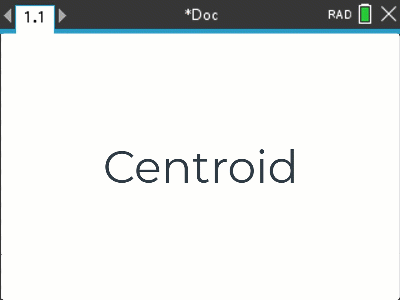•• ##### Device
•TI-Nspire™ CX
•TI-Nspire™ CX CAS
• ##### Software

TI-Nspire™
TI-Nspire™ CAS

ACMMG243
ACMNA234
ACMNA237

5.2

# Year 10A: Centroid

by Texas Instruments#### Objectives

The aim of this activity is to put a range of coordinate geometry skills into context, including midpoints, equations to lines (given two points) and simultaneous equations. The mixture of skills and processes helps students build and connect these skills.

#### Vocabulary

• Midpoint
• Straight line equation
• Simultaneous equations
• Distance between two points
• Similar triangles
• Geometric proof